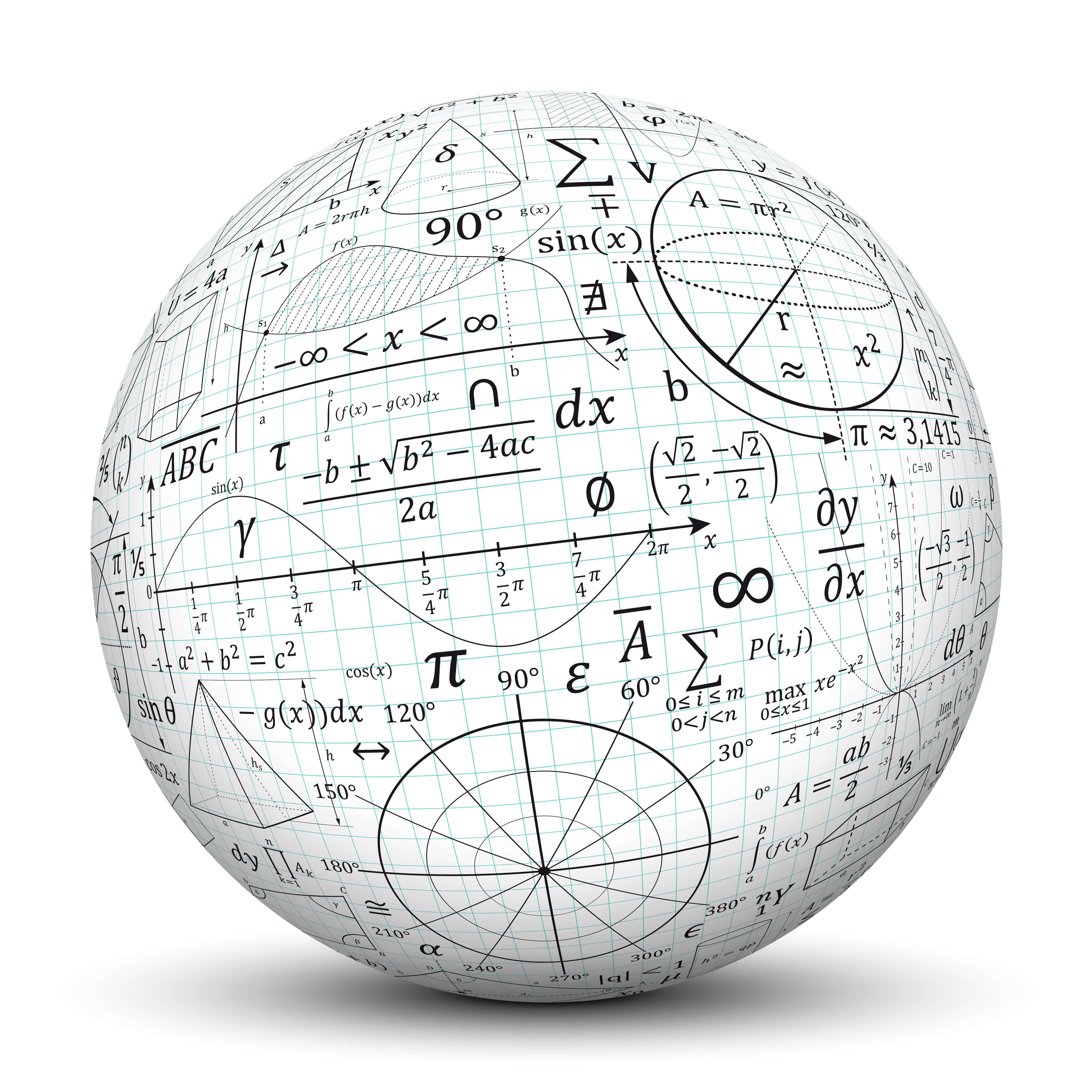# Mathematics - BScThe Bachelor of Science in mathematics develops a level of skill that enables students to apply their knowledge in industry or teaching, as well as prepare the student for advanced study of mathematics and other fields.

Graduates of the mathematics program will be able to:

• Apply their knowledge in the modern industry or teaching, or secure acceptance in high-quality graduate programs in mathematics and other fields such as the field of quantitative/mathematical finance, mathematical computing, statistics, and actuarial science

• Communicate effectively both orally and in writing

• Work effectively in teams

• Exhibit ethical and professional behavior

• Formulate and analyze mathematical and statistical problems, precisely define the key terms, and draw clear and reasonable conclusions

• Use mathematical and statistical techniques to solve well-defined problems and present their mathematical work, both in oral and written format, to various audiences (students, mathematicians, and non-mathematicians)

• Read, understand and construct correct mathematical and statistical proofs and use the library and electronic databases to locate information on mathematical problems

• Explain the importance of mathematics and its techniques to solve real-life problems and provide the limitations of such techniques and the validity of the results

• Propose new mathematical and statistical questions and suggest possible software packages and/or computer programming to find solutions to these questions

• Continue to acquire mathematical and statistical knowledge and skills appropriate to professional activities and demonstrate the highest standards of ethical issues in mathematics

As an option within the bachelor's degree in mathematics, students may choose to study statistics and data analysis.

Students intending to double-major in mathematics together with another program from the School of Sciences and Engineering will be allowed to transfer and double-count credits earned in approved courses from their program toward requirements for a major in mathematics. This will normally allow them to complete the two programs by adding between nine and 30 credits to the total required by their “other” major, depending on what this other major is and on how many general electives a particular student is required to take.

For double-major actuarial science/mathematics, consult with Zeinab Amin: zeinabha@aucegypt.edu